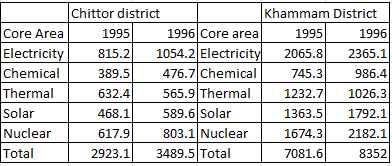### CAT 1996 Question Paper Question 63

Instructions

Answer the questions based on the following information. The amount of money invested (rupees in crores) in the core infrastructure areas of two districts, Chittoor and Khammam, Andhra Pradesh, is as follows.Question 63

# In Khammam district, the investment in which area in 1996 showed the highest percentage increase over the investment in that area in 1995?

Solution

In Electricity, the increase in investment is 300, which is less that 15%.

In Chemical, the increase is 241.1, which is greater than 32%.

In Thermal, there was a decrease in investment.

In Solar, the increase is 428.6, which is less than 32%.

In Nuclear, the increase in investment is 507.8, which is less than 31%.

Hence, the investment is the highest in chemical core area.

• All Quant CAT Formulas and shortcuts PDF
• 30+ CAT previous papers with solutions PDF